# Civil Engineering - Strength of Materials

### Exercise :: Strength of Materials - Section 1

21.

The minimum number of rivets for the connection of a gusset plate, is

 A. 1 B. 2 C. 3 D. 4

Explanation:

No answer description available for this question. Let us discuss.

22.

A triangular section having base b, height h, is placed with its base horizontal. If the shear stress at a depth y from top is q, the maximum shear stress is

 A.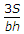B.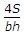C.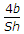D.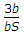Explanation:

No answer description available for this question. Let us discuss.

23.

The slenderness ratio of a vertical column of a square cross-section of 2.5 cm sides and 300 cm length, is

 A. 200 B. 240 C. 360 D. 416 E. 500

Explanation:

No answer description available for this question. Let us discuss.

24.

If n is the ratio of internal and external diameters of a hollow shaft, the ratio of the weight of the hollow shaft and that of solid shaft of same strength, will be

 A.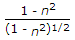B.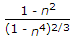C.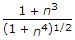D.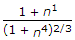Explanation:

No answer description available for this question. Let us discuss.

25.

A rectangular beam 20 cm wide is subjected to a maximum shearing force of 10, 000 kg, the corresponding maximum shearing stress being 30 kg/cm2. The depth of the beam is

 A. 15 cm B. 20 cm C. 25 cm D. 30 cm.